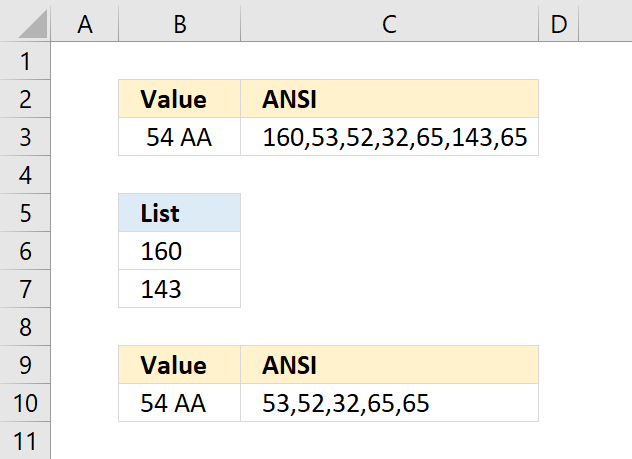Author: Oscar Cronquist Article last updated on April 20, 2022Cell B3 contains a few odd characters and the formula in C3 shows the ANSI equivalent of each character in B3. If you are interested in how I built the formula, read this: How to identify characters in a cell value

For example, 160 is an HTML space character that the TRIM and CLEAN function can't remove. You can remove a single character using the SUBSTITUTE function, however, if your cell contains multiple unwanted characters the following formula will remove specific characters.

Array formula in cell B10:

=TEXTJOIN(, TRUE, IF(COUNTIF(B6:B7, CODE(MID(B3, ROW(\$A\$1:INDEX(\$A\$1:\$A\$1000, LEN(B3))), 1))), "", MID(B3, ROW(\$A\$1:INDEX(\$A\$1:\$A\$1000, LEN(B3))), 1)))

The characters I want to remove are in B6:B7 in ANSI code format. The formula in cell C10 shows that 160 and 143 are now gone in cell B10.

### Explaining formula in cell B10

LEN(B3)

#### Step 2 - Create a cell reference

INDEX(\$A\$1:\$A\$1000, LEN(B3))

#### Step 3 - Create a cell range reference

The INDEX function and the LEN function allow you to create a cell reference containing as many rows as there are characters in cell B3.

\$A\$1:INDEX(\$A\$1:\$A\$1000, LEN(B3))

becomes

\$A\$1:INDEX(\$A\$1:\$A\$1000, 7)

and returns \$A\$1:\$A\$7.

#### Step 4 - Create a sequential list of numbers from 1 to n

The ROW function then creates an array from 1 to the number of characters in cell C3.

ROW(\$A\$1:INDEX(\$A\$1:\$A\$1000, LEN(B3)))

becomes

ROW(\$A\$1:\$A\$7)

and returns {1;2;3;4;5;6;7}

#### Step 5 - Split characters into an array

Now it is time for the MID function to split each character in cell C3 to an array.

MID(B3, ROW(\$A\$1:INDEX(\$A\$1:\$A\$1000, LEN(B3))), 1)

becomes

MID(B3, {1;2;3;4;5;6;7}) and returns {" ";"5";"4";" ";"A";"";"A"}

#### Step 6 - Convert characters to equivalent ANSI numbers

The CODE function allows you to convert a character to ANSI code (PC). We are using an array so the function will convert all characters at the same time.

CODE(MID(B3, ROW(\$A\$1:INDEX(\$A\$1:\$A\$1000, LEN(B3))), 1))

becomes

CODE({" ";"5";"4";" ";"A";"";"A"})

and returns {160;53;52;32;65;143;65}

#### Step 7 - Check ANSI numbers against list

The COUNTIF function counts how many times 160 and 143 are found in the array.

COUNTIF(B6:B7, CODE(MID(B3, ROW(\$A\$1:INDEX(\$A\$1:\$A\$1000, LEN(B3))), 1)))

becomes

COUNTIF({160;143},  {160;53;52;32;65;143;65})

and returns {1;0;0;0;0;1;0}

#### Step 8 - Filter values

The IF function checks the logical expression and returns a blank value if TRUE and the character if FALSE. The IF function evaluates TRUE as 1 and FALSE as 0 (zero).

IF(COUNTIF(B6:B7, CODE(MID(B3, ROW(\$A\$1:INDEX(\$A\$1:\$A\$1000, LEN(B3))), 1))), "", MID(B3, ROW(\$A\$1:INDEX(\$A\$1:\$A\$1000, LEN(B3))), 1))

becomes

IF({1;0;0;0;0;1;0},"",{" ";"5";"4";" ";"A";"";"A"})

and returns {"";"5";"4";" ";"A";"";"A"}.

#### Step 9 - Join characters

Lastly, the TEXTJOIN function concatenates the remaining characters in the array ignoring blank values.

TEXTJOIN(, TRUE, IF(COUNTIF(B6:B7, CODE(MID(B3, ROW(\$A\$1:INDEX(\$A\$1:\$A\$1000, LEN(B3))), 1))), "", MID(B3, ROW(\$A\$1:INDEX(\$A\$1:\$A\$1000, LEN(B3))), 1)))

becomes

TEXTJOIN(, TRUE, {"";"5";"4";" ";"A";"";"A"})

and returns 54 AA in cell B10.

### Get Excel *.xlsx file

How to remove unwanted characters from cell value.xlsx

## 2. How to remove unwanted characters in a cell - Excel 365 formulaExcel 365 dynamic array formula in cell B10:

=LET(x,MID(B3, SEQUENCE(LEN(B3)),1),TEXTJOIN(,TRUE,IF(COUNTIF(B6:B7,CODE(x)),"",x)))

### Explaining formula

LEN(B3)

#### Step 2 - Create a sequential list from 1 to n

SEQUENCE(LEN(B3))

#### Step 3 - Split characters into an array

Now it is time for the MID function to split each character in cell C3 to an array.

MID(B3, SEQUENCE(LEN(B3)), 1)

becomes

MID(B3, {1;2;3;4;5;6;7}) and returns {" ";"5";"4";" ";"A";"";"A"}

#### Step 4 - Convert characters to equivalent ANSI numbers

The CODE function allows you to convert a character to ANSI code (PC). We are using an array so the function will convert all characters at the same time.

CODE(MID(B3, SEQUENCE(LEN(B3)), 1))

becomes

CODE({" ";"5";"4";" ";"A";"";"A"})

and returns

{160;53;52;32;65;143;65}

#### Step 5 - Check ANSI numbers against list

The COUNTIF function counts how many times 160 and 143 are found in the array.

COUNTIF(B6:B7, CODE(MID(B3, SEQUENCE(LEN(B3)), 1)))

becomes

COUNTIF({160;143},  {160;53;52;32;65;143;65})

and returns

{1;0;0;0;0;1;0}

#### Step 6 - Filter values

The IF function checks the logical expression and returns a blank value if TRUE and the character if FALSE. The IF function evaluates TRUE as 1 and FALSE as 0 (zero).

IF(COUNTIF(B6:B7, CODE(MID(B3, SEQUENCE(LEN(B3)), 1))), "", MID(B3, SEQUENCE(LEN(B3)), 1))

becomes

IF({1;0;0;0;0;1;0},"",{" ";"5";"4";" ";"A";"";"A"})

and returns

{"";"5";"4";" ";"A";"";"A"}.

#### Step 7 - Join characters

The TEXTJOIN function concatenates the remaining characters in the array ignoring blank values.

TEXTJOIN(, TRUE, IF(COUNTIF(B6:B7, CODE(MID(B3, SEQUENCE(LEN(B3)), 1))), "", MID(B3, SEQUENCE(LEN(B3)), 1)))

becomes

TEXTJOIN(, TRUE, {"";"5";"4";" ";"A";"";"A"})

and returns 54 AA

#### Step 8 - Simplify formula

The LET function lets you name intermediate calculation results which can shorten formulas considerably and improve performance.

LET(name1name_value1calculation_or_name2, [name_value2calculation_or_name3...])

TEXTJOIN(, TRUE, IF(COUNTIF(B6:B7, CODE(MID(B3, SEQUENCE(LEN(B3)), 1))), "", MID(B3, SEQUENCE(LEN(B3)), 1)))

has two repeating intermediate calculations bolded in the formula above:

MID(B3, SEQUENCE(LEN(B3)), 1) - x

I named it x.

LET(x,MID(B3, SEQUENCE(LEN(B3)),1),TEXTJOIN(,TRUE,IF(COUNTIF(B6:B7,CODE(x)),"",x)))